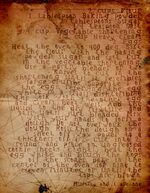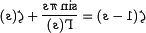Riemann HypothesisHere is the original manuscript of the Artist Formerly Known As "Opty Cool R."

The Riemann Hypothesis was a key mathematical conjecture, in the field of theoretical gangsta rap, which remained unsolved for centuries. It concerns the behavior of the quality function in any given song. This predicts where and when the song will hit rock bottom (or zero, as mathematicians call it, to appear smarter than they really are). Of course, as it regards rap music, the quality function is an imaginary number.

The importance of this conjecture for number theory and cryptography led the Clay Institute to offer a million-dollar prize for its solution. It was finally proven in 2004 by famous logician, mathematician and finger-painter Chuck Norris. His remarkably simple and direct proof is presented below.

History

For many years, mathematicians puzzled over the hypothesis, first presented in the early 1980s by Optimus "Riemann" Rhyme — none other than the original "Rhyme-man."

In one of its equivalent formulations, it states that:

“No matter which word you end a line of a song with, there will always exist a word that rhymes with it for use in future lines.”

~ Rhymin' Opty's formulation of the Riemann Hypothesis

This was obviously of great importance back in the days of "old skool" rap and is responsible for Opty's meteoric rise to fame. However, songwriters are still looking for the word that rhymes with rainbow.

Skeptics

Attempts to disprove the Riemann Hypothesis began in 1981 when The Sugarhill Gang released a controversial track with the ending line, "Like an orange." This clear challenge to the theory, from such prominent academics, took the world of mathematics by storm.

It wasn't until 1991 that the future-hit sensation De La Soul, citing the Rule of Three, released their smash hit, Orange, So Sorange, Why Don't You Get Off My Morange? Its meteoric rise to 9th place on the national rap charts showed that The Sugarhill Gang's challenge had been defeated. The formal proof, however, had to wait another decade for the analytical genius of Chuck Norris.

The Norris Proof of the Riemann HypothesisNo photographs of Riemann survive to the present. However, we know he was a German, so he may have looked something like this.

Norris's proof is set out briefly below; and let it never again be said that Norris's most notable attribute is his roundhouse kick. We assume the reader has basic knowledge of analytic number theory and pottery.

Theorem: In any single piece of rap music, the imaginary quality of the music is a function of how stoned the writer is, $\zeta (s)$. The quality hits rock bottom (zero) when the writer is exactly half stoned: too far out to produce coherent stuff, and too sober to access his subconscious genius.

Proof: In the begining, there is an L-function, which looks like this:

$\zeta (s)=\sum _{n}^{\infty }{\frac {L}{n^{s}}}$When L=1, then every zero has s=1/2 which can be shown using the following easy proof:

$\zeta (s+1)-\zeta (s)=\sum _{n}^{\infty }{\frac {1}{(n+1)^{s}}}-\sum _{n}^{\infty }{\frac {1}{n^{s}}}={\frac {1}{n^{s}}}$Substituting n=1/2 into the formula and solving for s, one gets:

$\zeta (s+1)-\zeta (s)=2^{s}$According to the reflection theorem, one has:

$\zeta (1-s)={\frac {\sin \pi s}{\Gamma (s)}}+\zeta (s)$and upon further reflection, one obtains:which means:

$\zeta (s+1)={\sqrt {2}}+\zeta (s)$Substituting into the above means that:

${\sqrt {2}}=2^{s}$Thus, s=1/2. This is the easiest proof of the Riemann Hypothesis, but it can be proven in other ways, which are left as an exercise to the reader.

Effects

In 1900, David Hilbert included the Riemann Hypothesis in his list of the 23 simplest and least mathematically significant problems.